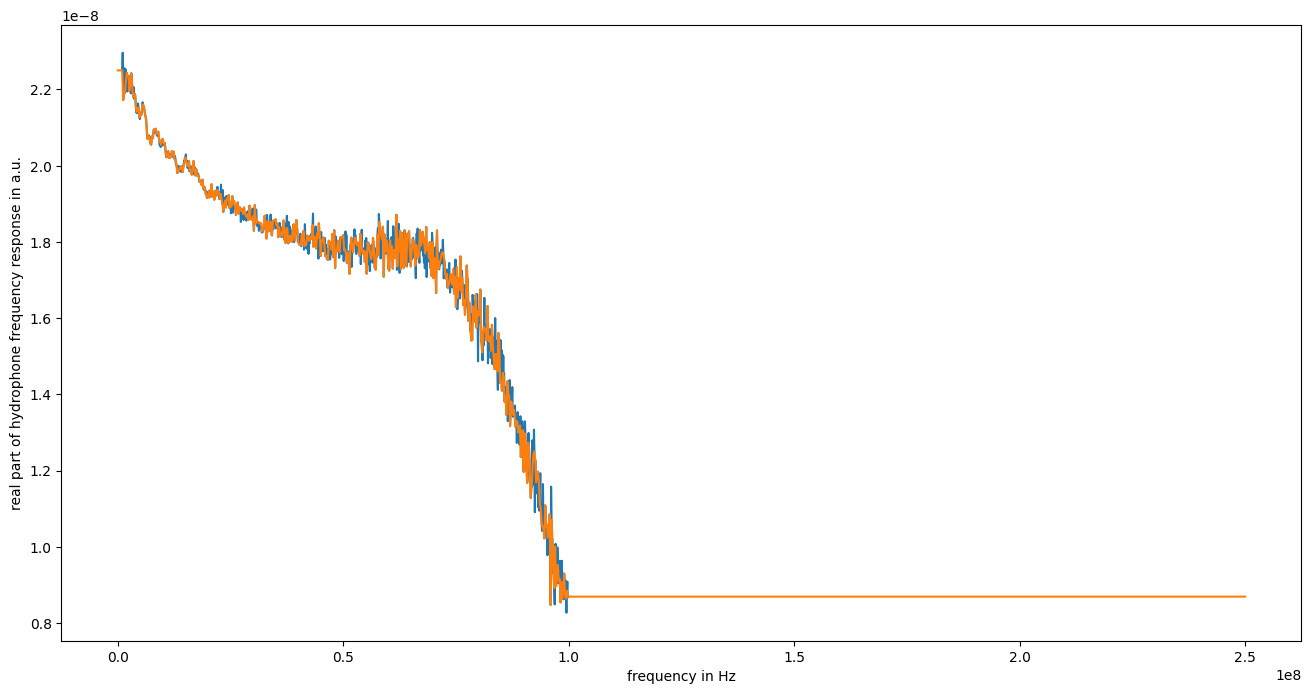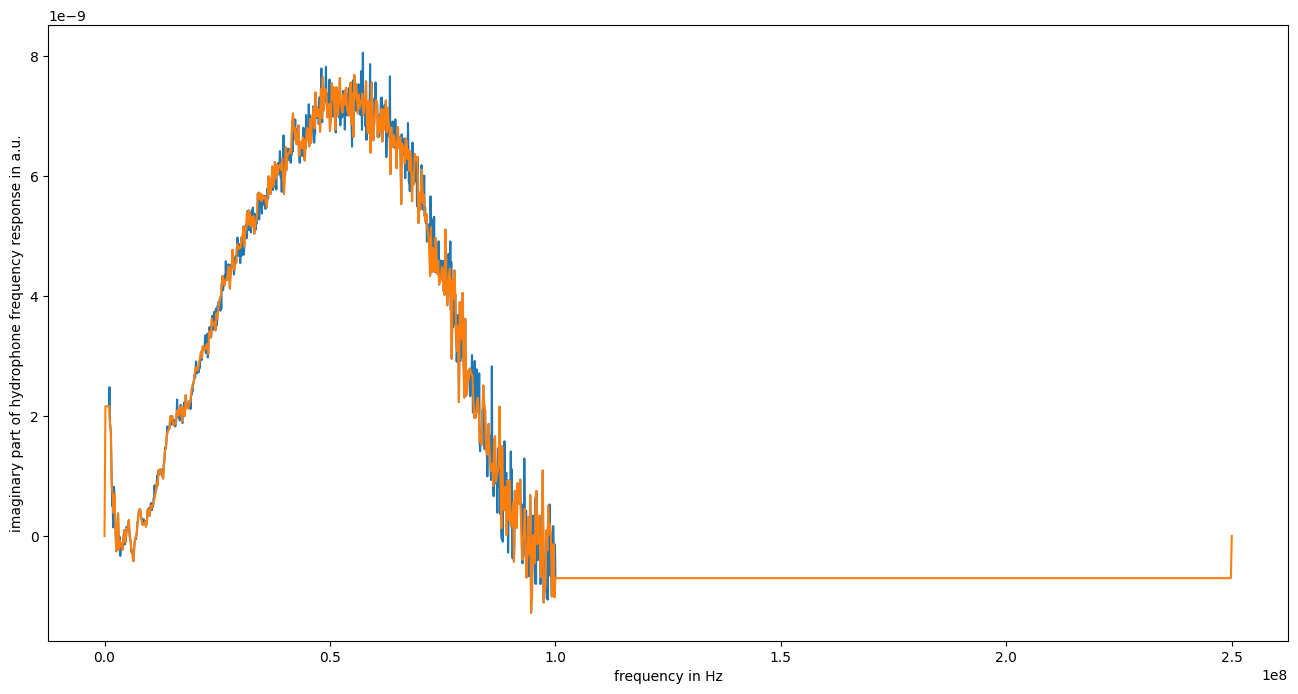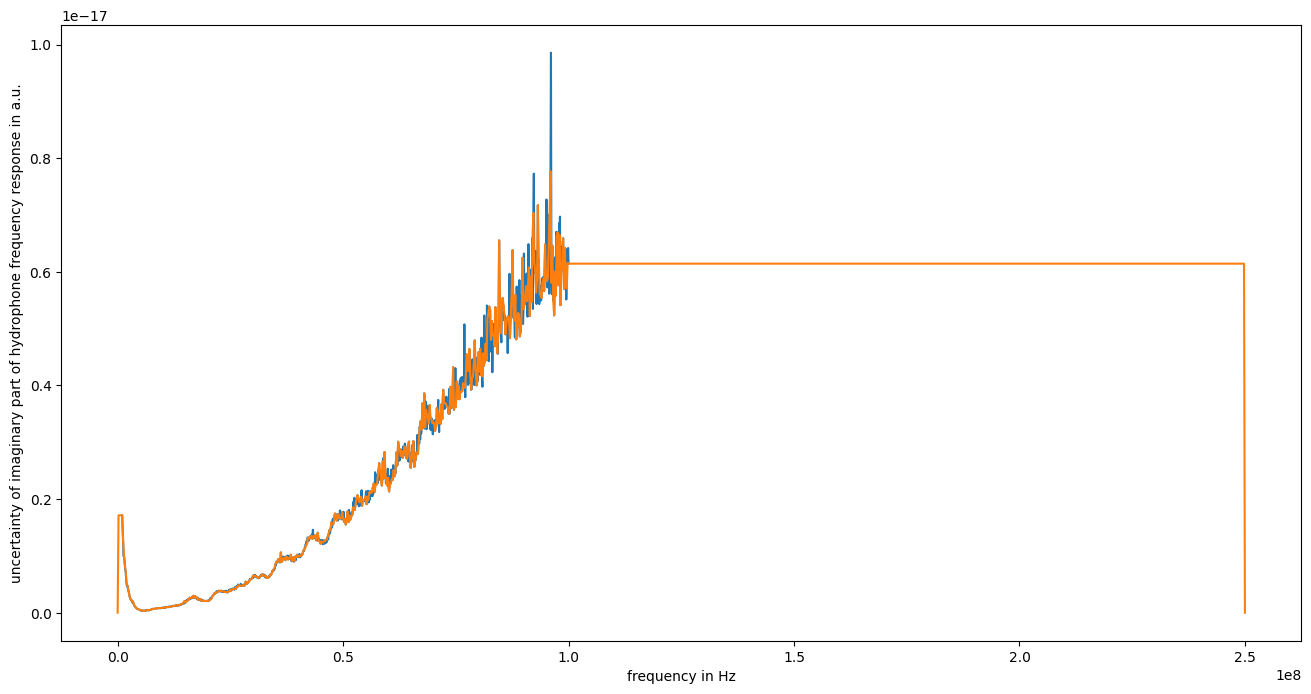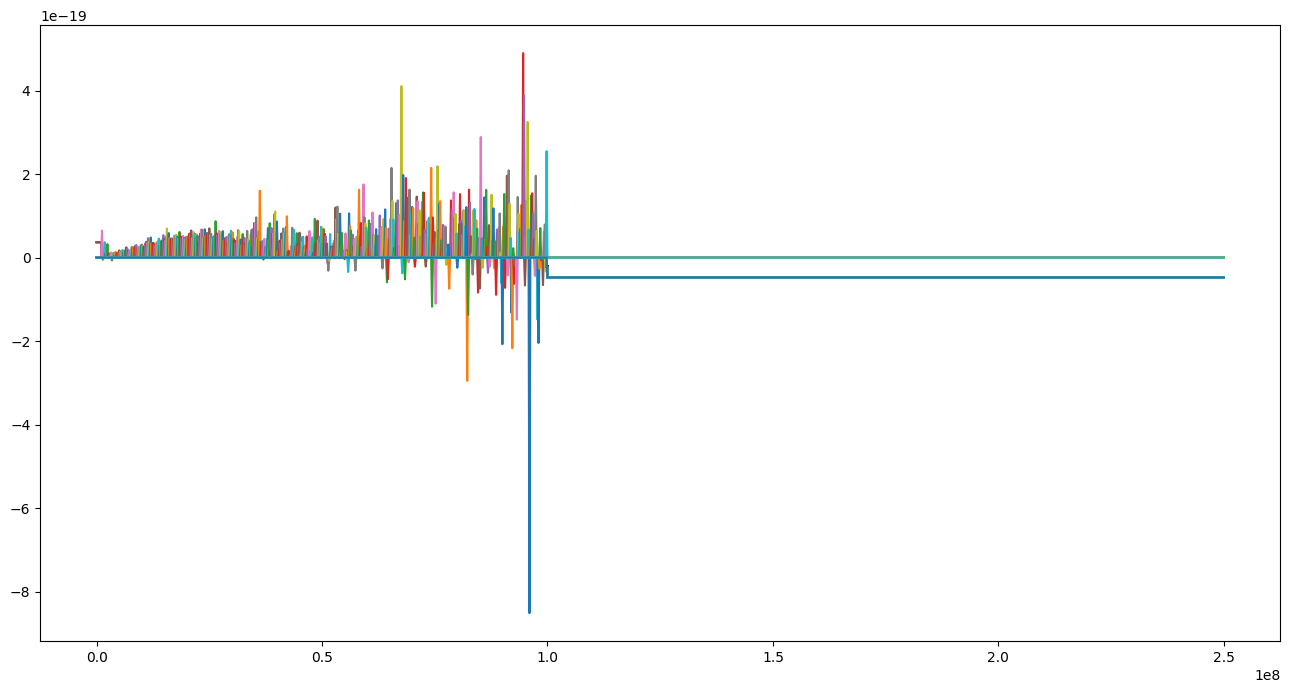# 3. Interpolation and extrapolation of calibration data¶

:

%pylab inline

Populating the interactive namespace from numpy and matplotlib

:

from meas_data_preprocessing import *
from hydrophone_data_preprocessing import *

/home/ludwig10/code/envs/PyDynamic_tutorials-py38/lib/python3.8/site-packages/PyDynamic/identification/fit_filter.py:24: DeprecationWarning: The module *identification* will be combined with the module *deconvolution* and renamed to *model_estimation* in the next major release 2.0.0. From version 1.4.1 on you should only use the new module *model_estimation* instead.
warnings.warn(
/home/ludwig10/code/envs/PyDynamic_tutorials-py38/lib/python3.8/site-packages/PyDynamic/identification/fit_transfer.py:23: DeprecationWarning: The package *identification* will be combined with the package *deconvolution* and renamed to *model_estimation* in the next major release 2.0.0. From version 1.4.1 on you should only use the new package *model_estimation* instead.
warnings.warn(
/home/ludwig10/code/envs/PyDynamic_tutorials-py38/lib/python3.8/site-packages/PyDynamic/uncertainty/interpolation.py:24: PendingDeprecationWarning: The module :mod:PyDynamic.uncertainty.interpolation will be renamed to :mod:PyDynamic.uncertainty.interpolate in the next major release 2.0.0. From version 1.4.3 on you should only use the new module instead.
warnings.warn(

:

from PyDynamic.uncertainty.interpolation import interp1d_unc

/home/ludwig10/code/envs/PyDynamic_tutorials-py38/lib/python3.8/site-packages/ipykernel/ipkernel.py:283: DeprecationWarning: should_run_async will not call transform_cell automatically in the future. Please pass the result to transformed_cell argument and any exception that happen during thetransform in preprocessing_exc_tuple in IPython 7.17 and above.
and should_run_async(code)


## Read measured data and calibration data from file¶

:

meas_scenario = 13
infos, measurement_data = read_data(meas_scenario = meas_scenario)
_, hyd_data = read_calib_data(meas_scenario = meas_scenario, do_plot = False)

Checking if file ../datasets/pD7_MH44.DAT is already present or download it from https://raw.githubusercontent.com/Ma-Weber/Tutorial-Deconvolution/master/MeasuredSignals/pD-Mode%207%20MHz/pD7_MH44.DAT otherwise:
Replace is False and data exists, so doing nothing. Use replace=True to re-download the data.
Replace is False and data exists, so doing nothing. Use replace=True to re-download the data.
Replace is False and data exists, so doing nothing. Use replace=True to re-download the data.
The file ../datasets/pD7_MH44.DAT was read and it contains 2500 data points.
The time increment is 2e-09 s
Replace is False and data exists, so doing nothing. Use replace=True to re-download the data.
Replace is False and data exists, so doing nothing. Use replace=True to re-download the data.
Replace is False and data exists, so doing nothing. Use replace=True to re-download the data.

:

# metadata for chosen measurement scenario
for key in infos.keys():
print("%20s: %s" %(key,infos[key]))

                   i: 13
hydrophonname: GAMPT MH44
measurementtype: Pulse-Doppler-Mode 7 MHz
measurementfile: ../datasets/pD7_MH44.DAT
noisefile: ../datasets/pD7_MH44r.DAT
hydfilename: ../datasets/MW_MH44ReIm.csv


## Perform basic pre-processing¶

:

# remove DC component in measured data
measurement_data = remove_DC_component(measurement_data)

:

# reduce frequency range of calibration data
hyd_data = reduce_freq_range(hyd_data, fmin = 1e6, fmax = 100e6)


## Align spectral data of calibration and measured data¶

:

measurement_data = uncertainty_from_noisefile(infos, measurement_data, do_plot=False, verbose=False)
measurement_data = calculate_spectrum(measurement_data, do_plot = False)
fmeas = measurement_data["frequency"].round()
N = len(fmeas)//2


### Interpolation of real part¶

:

hyd_interp = dict([])
hyd_interp["frequency"], hyd_interp["real"], hyd_interp["varreal"], Creal = interp1d_unc(
fmeas[:N], hyd_data["frequency"], hyd_data["real"], hyd_data["varreal"],
bounds_error=False, fill_value="extrapolate", fill_unc="extrapolate", returnC=True)

:

figure(figsize=(16,8))
plot(hyd_data["frequency"], hyd_data["real"])
plot(hyd_interp["frequency"], hyd_interp["real"])
xlabel("frequency in Hz")
ylabel("real part of hydrophone frequency response in a.u.")

figure(figsize=(16,8))
plot(hyd_data["frequency"], hyd_data["varreal"])
plot(hyd_interp["frequency"], hyd_interp["varreal"])
xlabel("frequency in Hz")
ylabel("uncertainty of real part of hydrophone frequency response in a.u.");### Interpolation of imaginary part¶

:

hyd_interp["frequency"], hyd_interp["imag"], hyd_interp["varimag"], Cimag = interp1d_unc(
fmeas[:N], hyd_data["frequency"], hyd_data["imag"], hyd_data["varimag"],
bounds_error=False, fill_value="extrapolate", fill_unc="extrapolate", returnC=True)
hyd_interp["imag"] = 0  # Must be 0 by definition
hyd_interp["imag"][-1] = 0
hyd_interp["varimag"] = 0  # Must be 0 by definition
hyd_interp["varimag"][-1] = 0

/home/ludwig10/code/envs/PyDynamic_tutorials-py38/lib/python3.8/site-packages/ipykernel/ipkernel.py:283: DeprecationWarning: should_run_async will not call transform_cell automatically in the future. Please pass the result to transformed_cell argument and any exception that happen during thetransform in preprocessing_exc_tuple in IPython 7.17 and above.
and should_run_async(code)

:

figure(figsize=(16,8))
plot(hyd_data["frequency"], hyd_data["imag"])
plot(hyd_interp["frequency"], hyd_interp["imag"])
xlabel("frequency in Hz")
ylabel("imaginary part of hydrophone frequency response in a.u.")

figure(figsize=(16,8))
plot(hyd_data["frequency"], hyd_data["varimag"])
plot(hyd_interp["frequency"], hyd_interp["varimag"])
xlabel("frequency in Hz")
ylabel("uncertainty of imaginary part of hydrophone frequency response in a.u.");### Calculation of mixed uncertainties at new frequencies¶

$U_{r_{interp},i_{interp}} = C_{r} U_{r,i} C_{i}^T$
:

hyd_interp["cov"] = (Creal.dot(diag(hyd_data["cov"]))).dot(Cimag.T)

/home/ludwig10/code/envs/PyDynamic_tutorials-py38/lib/python3.8/site-packages/ipykernel/ipkernel.py:283: DeprecationWarning: should_run_async will not call transform_cell automatically in the future. Please pass the result to transformed_cell argument and any exception that happen during thetransform in preprocessing_exc_tuple in IPython 7.17 and above.
and should_run_async(code)

:

figure(figsize=(16,8))
plot(hyd_interp["frequency"], hyd_interp["cov"]);